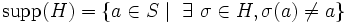# Weakly normal subgroup of symmetric group must move more than half the elements

Suppose$S$ is a set,$G = \operatorname{Sym}(S)$ is the Symmetric group (?) on$S$, and$H$ is a nontrivial Weakly normal subgroup (?) of$G$. Define:$\operatorname{supp}(H) = \{ a \in S \mid \ \exists \ \sigma \in H, \sigma(a) \ne a \}$.
Then, the cardinality of$\operatorname{supp}(H)$ should be strictly greater than the cardinality of$S \setminus \operatorname{supp}(H)$.